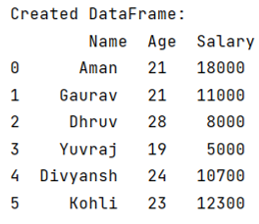# How to convert multiple lists into DataFrame?

Given multiple lists, we have to convert then into a Pandas DataFrame.
Submitted by Pranit Sharma, on May 28, 2022

Pandas is a special tool that allows us to perform complex manipulations of data effectively and efficiently. Inside pandas, we mostly deal with a dataset in the form of DataFrame. DataFrames are 2-dimensional data structures in pandas. DataFrames consist of rows, columns, and the data.

DataFrame can be created with the help of python dictionaries or lists but in the real world, CSV files are imported and then converted into DataFrames here we are going to learn how to convert multiple lists into a DataFrame.

There are many ways to achieve this task but the simplest way to do this is to create a final list consisting of all the lists which we want inside a DataFrame and then apply pandas.DataFrame() method.

Syntax:

```class pandas.DataFrame(
data=None,
index=None,
columns=None,
dtype=None,
copy=None
)
```

To work with pandas, we need to import pandas package first, below is the syntax:

```import pandas as pd
```

Let us understand with the help of an example.

```# Importing pandas package
import pandas as pd

# Creating an array
arr1 = ['Aman', 21, 18000]
arr2 = ['Gaurav', 21, 11000]
arr3 = ['Dhruv', 28, 8000]
arr4 = ['Yuvraj', 19, 5000]
arr5 = ['Divyansh', 24, 10700]
arr6 = ['Kohli', 23, 12300]

final_arr = [arr1,arr2,arr3,arr4,arr5,arr6]

# Creating a DataFrame
df = pd.DataFrame(final_arr, columns=['Name','Age','Salary'])

# Display created DataFrame
print("Created DataFrame:\n",df,"\n")
```

Output:Languages: » C » C++ » C++ STL » Java » Data Structure » C#.Net » Android » Kotlin » SQL
Web Technologies: » PHP » Python » JavaScript » CSS » Ajax » Node.js » Web programming/HTML
Solved programs: » C » C++ » DS » Java » C#
Aptitude que. & ans.: » C » C++ » Java » DBMS
Interview que. & ans.: » C » Embedded C » Java » SEO » HR
CS Subjects: » CS Basics » O.S. » Networks » DBMS » Embedded Systems » Cloud Computing
» Machine learning » CS Organizations » Linux » DOS
More: » Articles » Puzzles » News/Updates